This site uses cookies. By continuing to browse the ConceptDraw site you are agreeing to our Use of Site Cookies.
ConceptDraw Samples | Software Development — EXPRESS-G data Modeling Diagram

# EXPRESS-G data Modeling Diagram

The EXPRESS-G data Modeling diagrams are created using ConceptDraw DIAGRAM diagramming and vector drawing software enhanced with EXPRESS-G data Modeling Diagram solution from ConceptDraw Solution Park.

ConceptDraw DIAGRAM provides export of vector graphic multipage documents into multiple file formats: vector graphics (SVG, EMF, EPS), bitmap graphics (PNG, JPEG, GIF, BMP, TIFF), web documents (HTML, PDF), PowerPoint presentations (PPT), Adobe Flash (SWF).

## Tutorials and Solutions:

Video Tutorials: ConceptDraw Solution Park

Solutions: EXPRESS-G data Modeling Diagram for ConceptDraw DIAGRAM

## Sample 1: EXPRESS-G Data Modeling Diagram — Attribute Symbols

EXPRESS-G data Modeling Diagram sample: EXPRESS-G Data Modeling Diagram — Attribute Symbols.

This example is created using ConceptDraw DIAGRAM diagramming software enhanced with EXPRESS-G data Modeling Diagram solution from ConceptDraw Solution Park.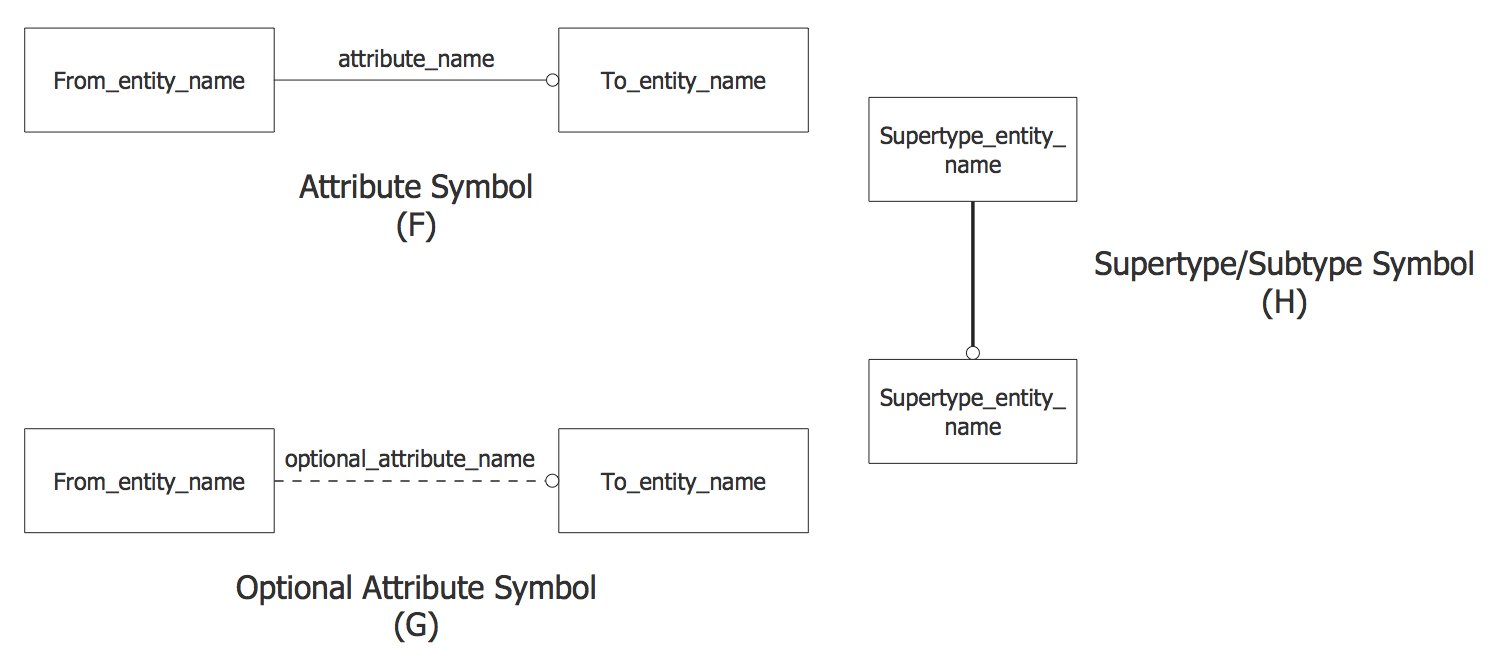## Sample 2: EXPRESS-G Data Type Symbols

EXPRESS-G data Modeling Diagram sample: EXPRESS-G Data Type Symbols.

This example is created using ConceptDraw DIAGRAM diagramming software enhanced with EXPRESS-G data Modeling Diagram solution from ConceptDraw Solution Park.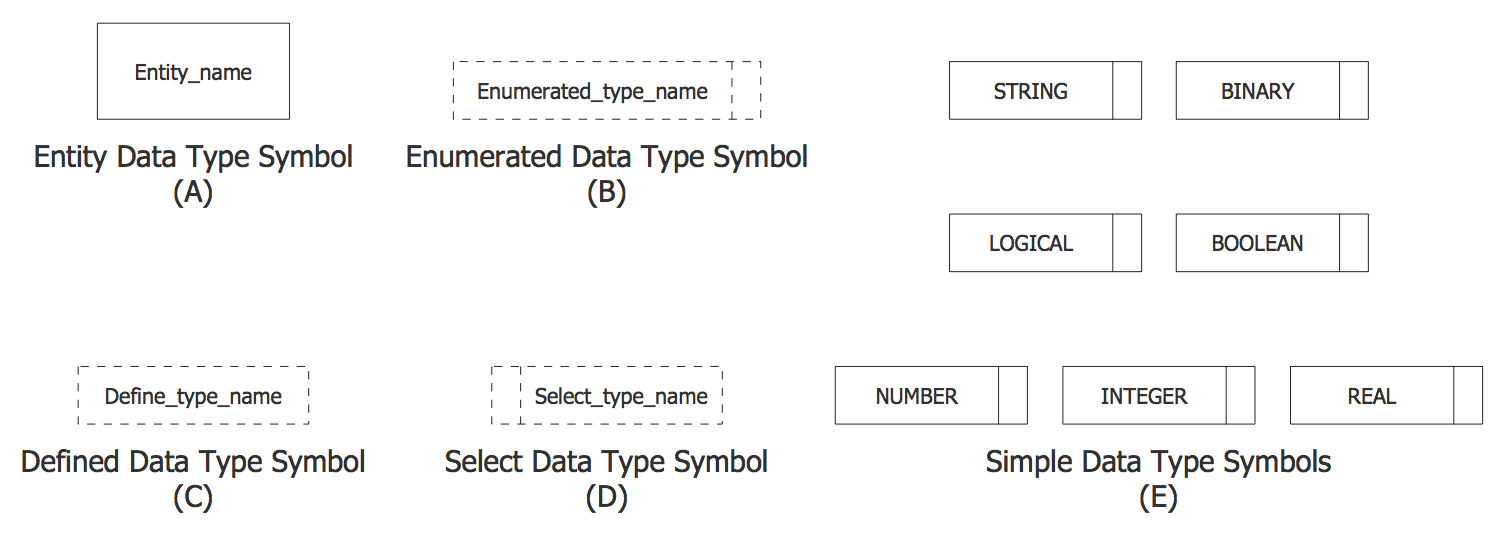## Sample 3: EXPRESS-G Diagram — Audio Compact Disc Collection

EXPRESS-G data Modeling Diagram sample: EXPRESS-G Diagram — Audio Compact Disc Collection.

This example is created using ConceptDraw DIAGRAM diagramming software enhanced with EXPRESS-G data Modeling Diagram solution from ConceptDraw Solution Park.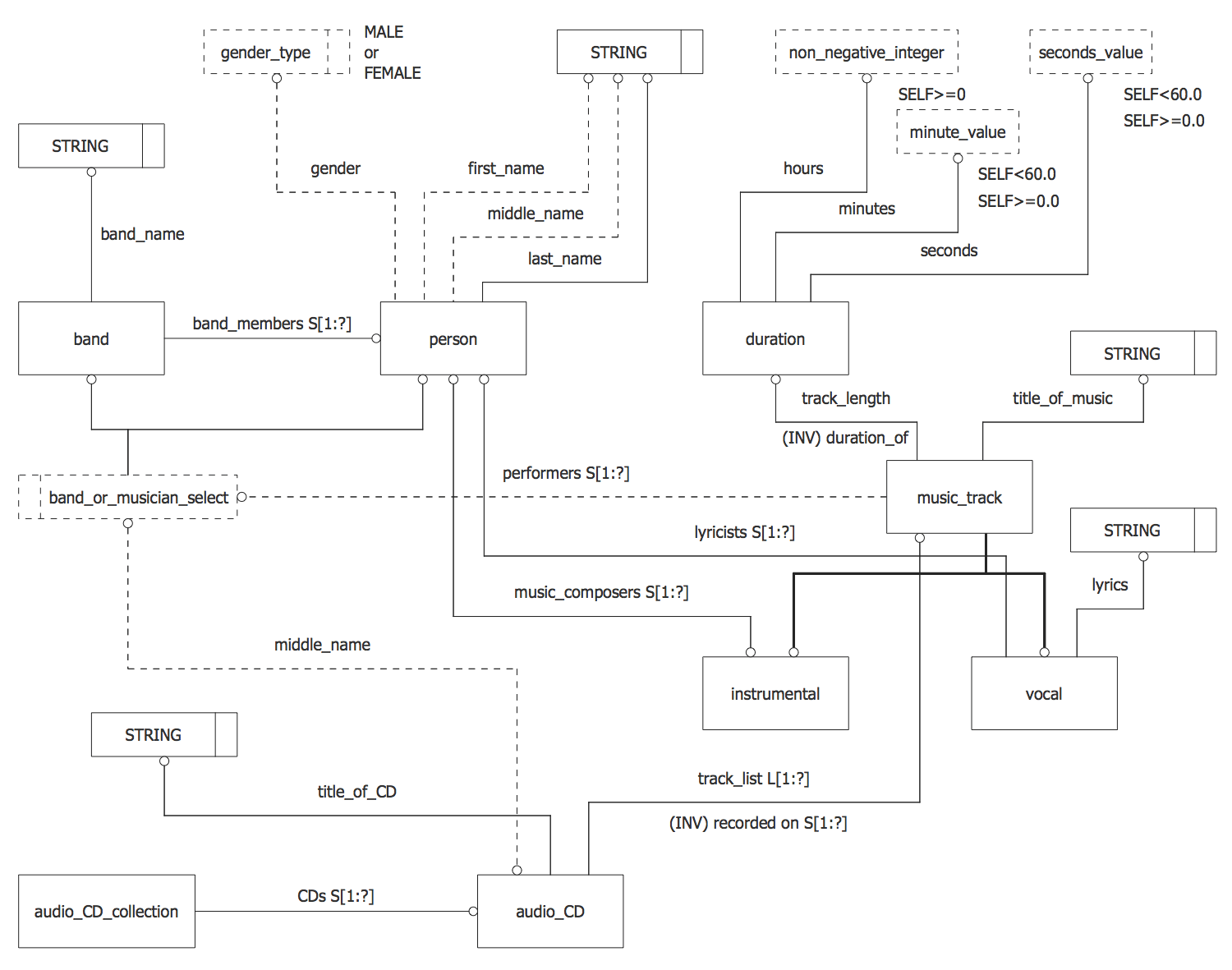## Sample 4: EXPRESS-G Diagram — DSCDM Datum Entity

EXPRESS-G data Modeling Diagram sample: EXPRESS-G Diagram — DSCDM Datum Entity.

This example is created using ConceptDraw DIAGRAM diagramming software enhanced with EXPRESS-G data Modeling Diagram solution from ConceptDraw Solution Park.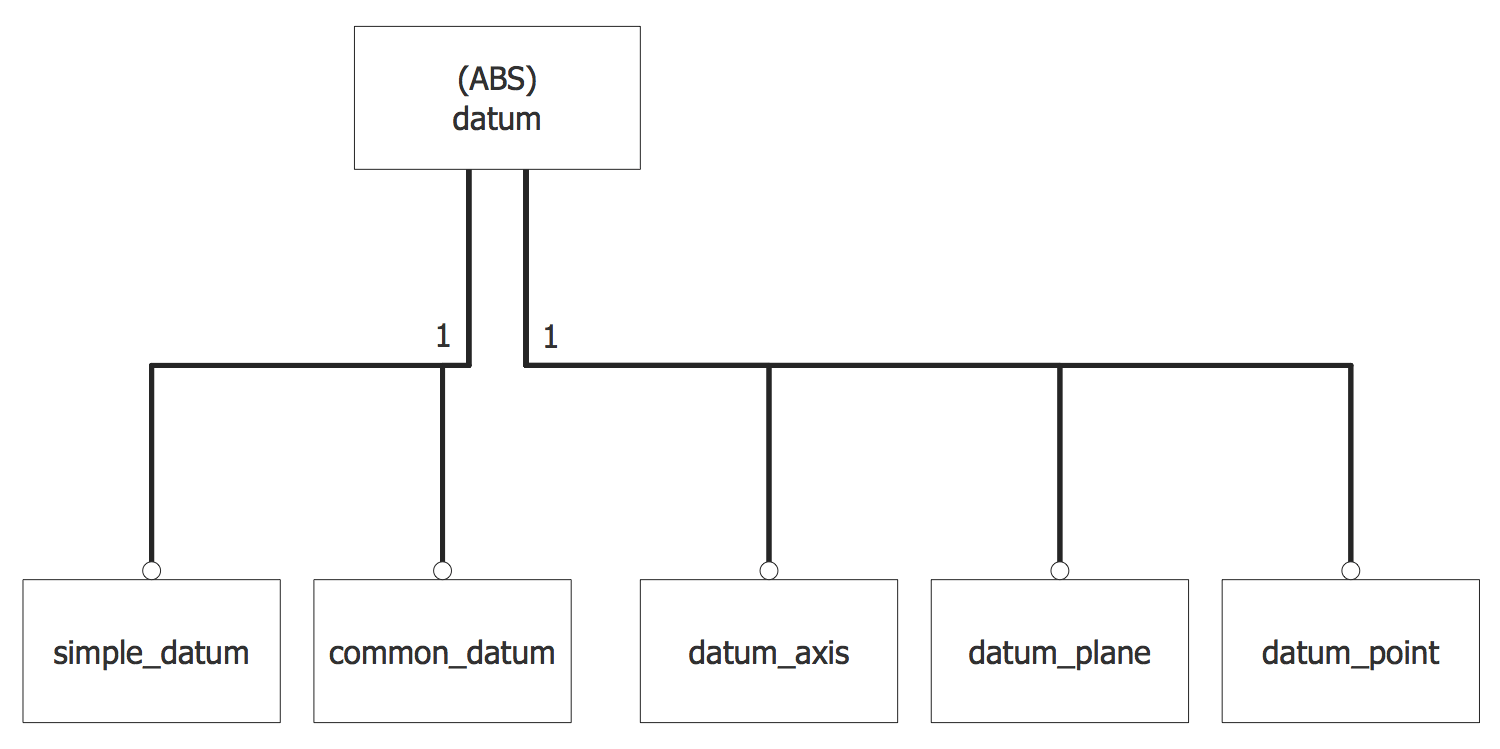## Sample 5: DSCDM Datum Feature and Datum Target Entities

EXPRESS-G data Modeling Diagram sample: DSCDM Datum Feature and Datum Target Entities.

This example is created using ConceptDraw DIAGRAM diagramming software enhanced with EXPRESS-G data Modeling Diagram solution from ConceptDraw Solution Park.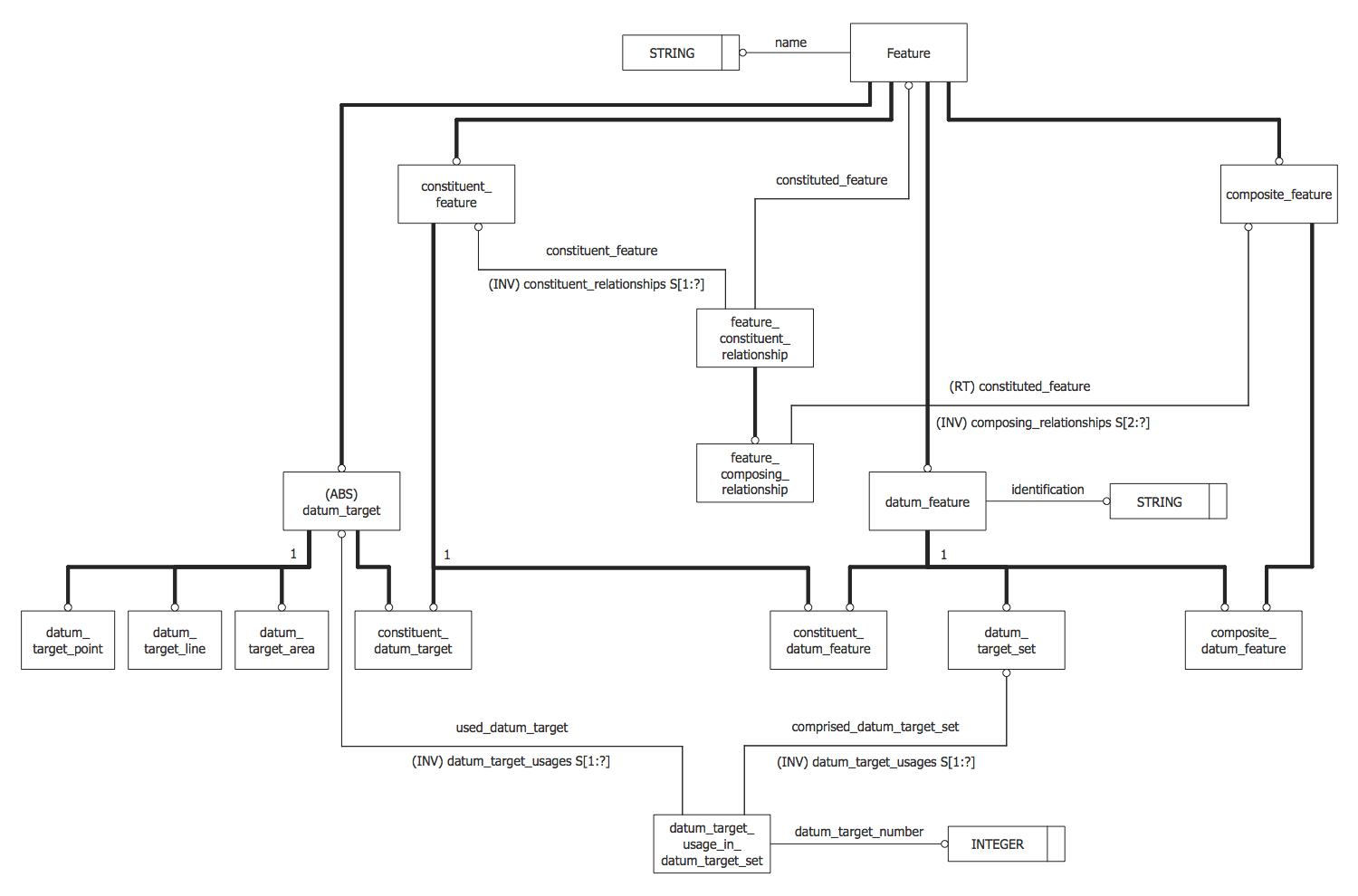## Sample 6: EXPRESS-G Diagram — Extended Version of the DSCDM

EXPRESS-G data Modeling Diagram sample: EXPRESS-G Diagram — Extended Version of the DSCDM.

This example is created using ConceptDraw DIAGRAM diagramming software enhanced with EXPRESS-G data Modeling Diagram solution from ConceptDraw Solution Park.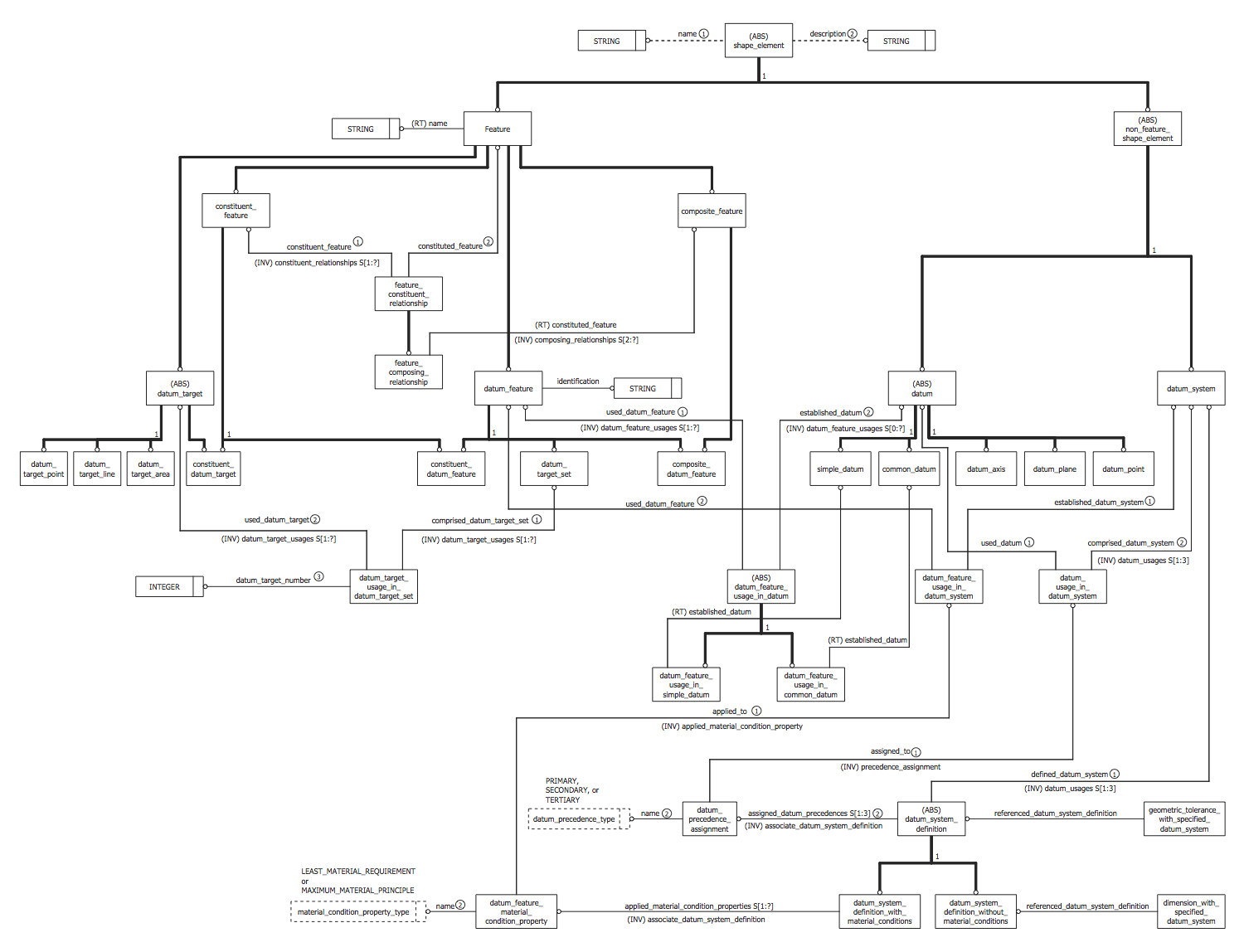## Sample 7: EXPRESS-G Diagram — Family Schema

EXPRESS-G data Modeling Diagram sample: EXPRESS-G Diagram — Family Schema.

This example is created using ConceptDraw DIAGRAM diagramming software enhanced with EXPRESS-G data Modeling Diagram solution from ConceptDraw Solution Park.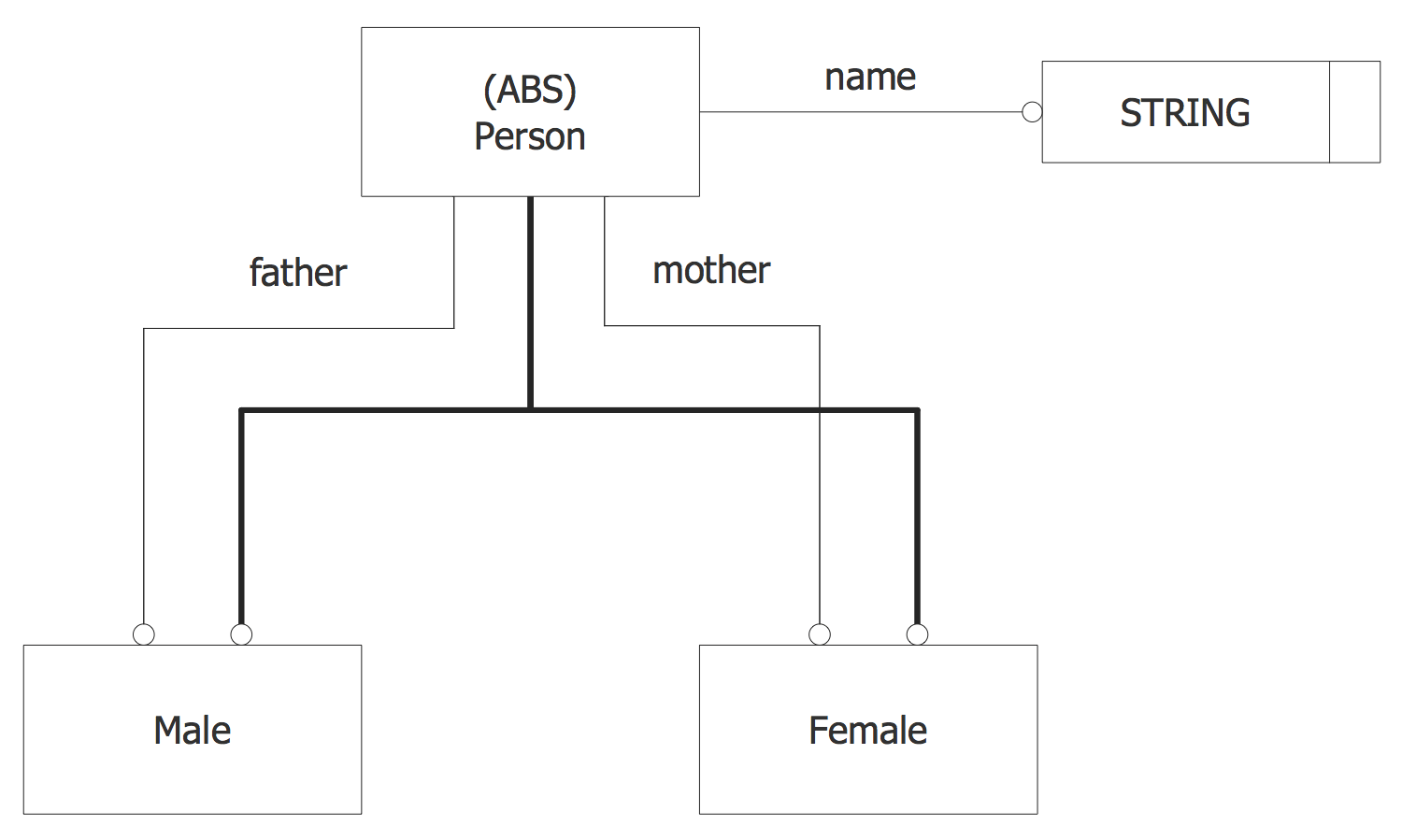## Sample 8: EXPRESS-G Diagram — STEP NC Process Model

EXPRESS-G data Modeling Diagram sample: EXPRESS-G Diagram — STEP NC Process Model.

This example is created using ConceptDraw DIAGRAM diagramming software enhanced with EXPRESS-G data Modeling Diagram solution from ConceptDraw Solution Park.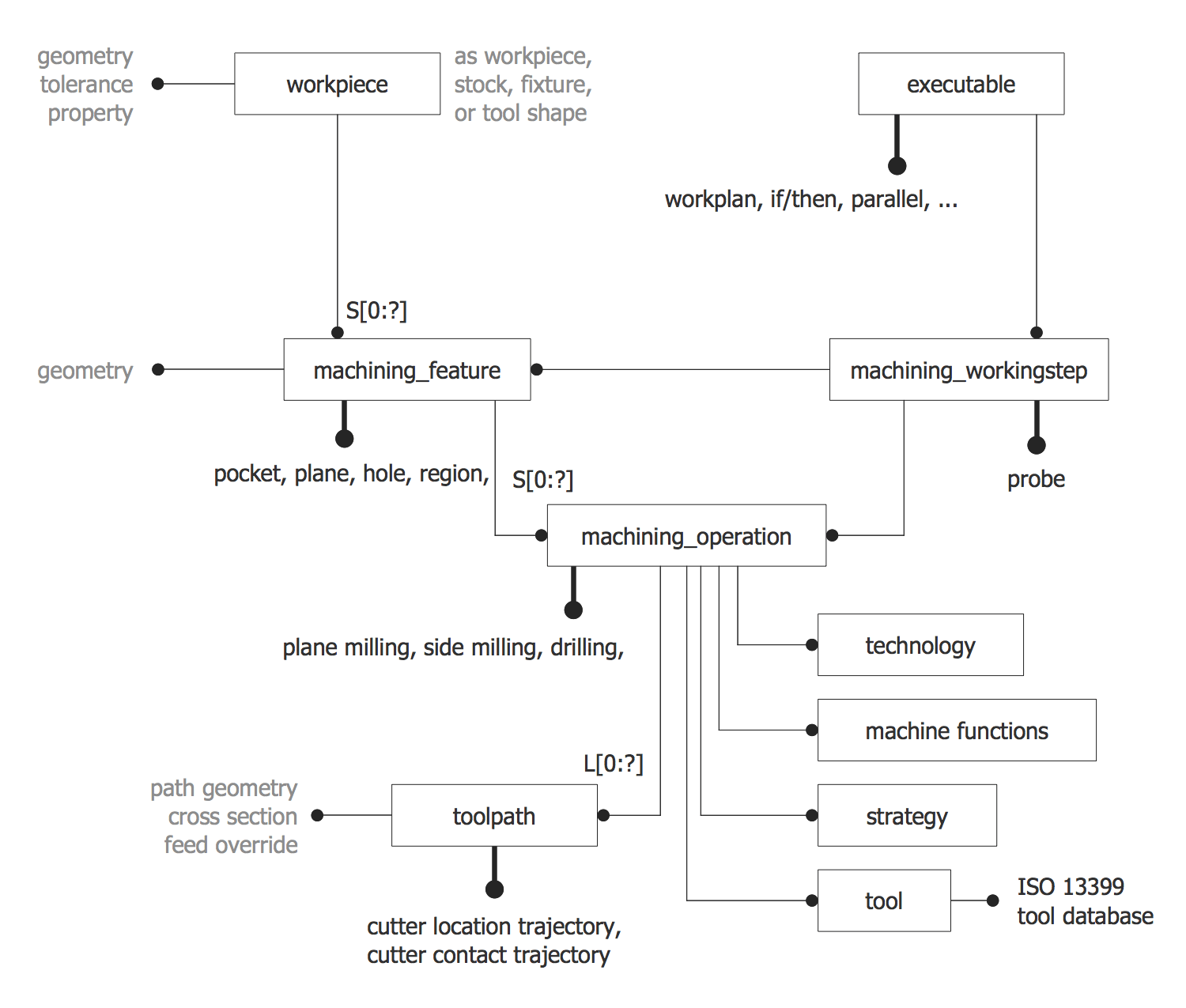## Sample 9: EXPRESS-G Model

EXPRESS-G data Modeling Diagram sample: EXPRESS-G Model.

This example is created using ConceptDraw DIAGRAM diagramming software enhanced with EXPRESS-G data Modeling Diagram solution from ConceptDraw Solution Park.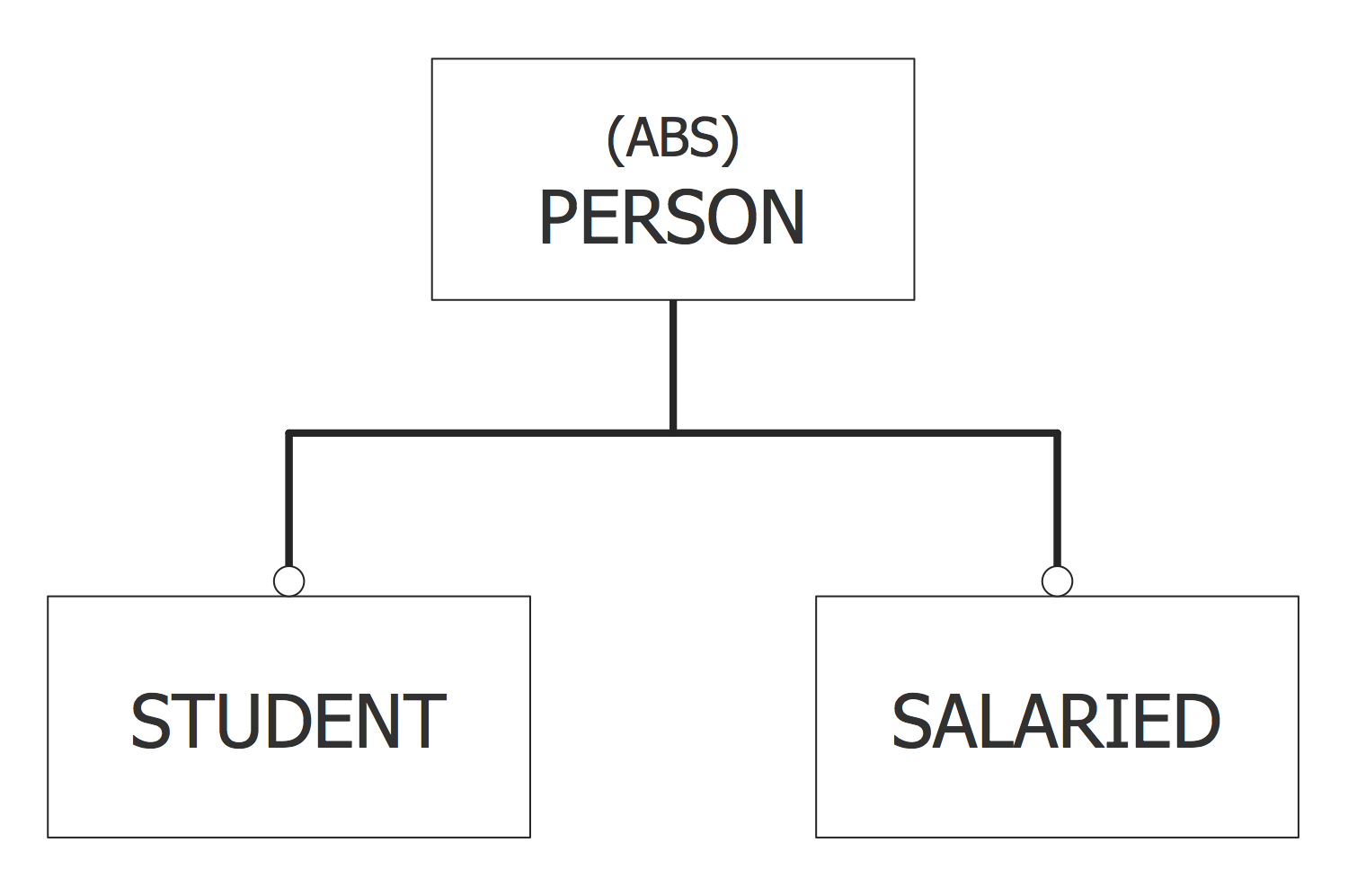## Sample 10: Symbols of the EXPRESS-G Notation

EXPRESS-G data Modeling Diagram sample: Symbols of the EXPRESS-G Notation.

This example is created using ConceptDraw DIAGRAM diagramming software enhanced with EXPRESS-G data Modeling Diagram solution from ConceptDraw Solution Park.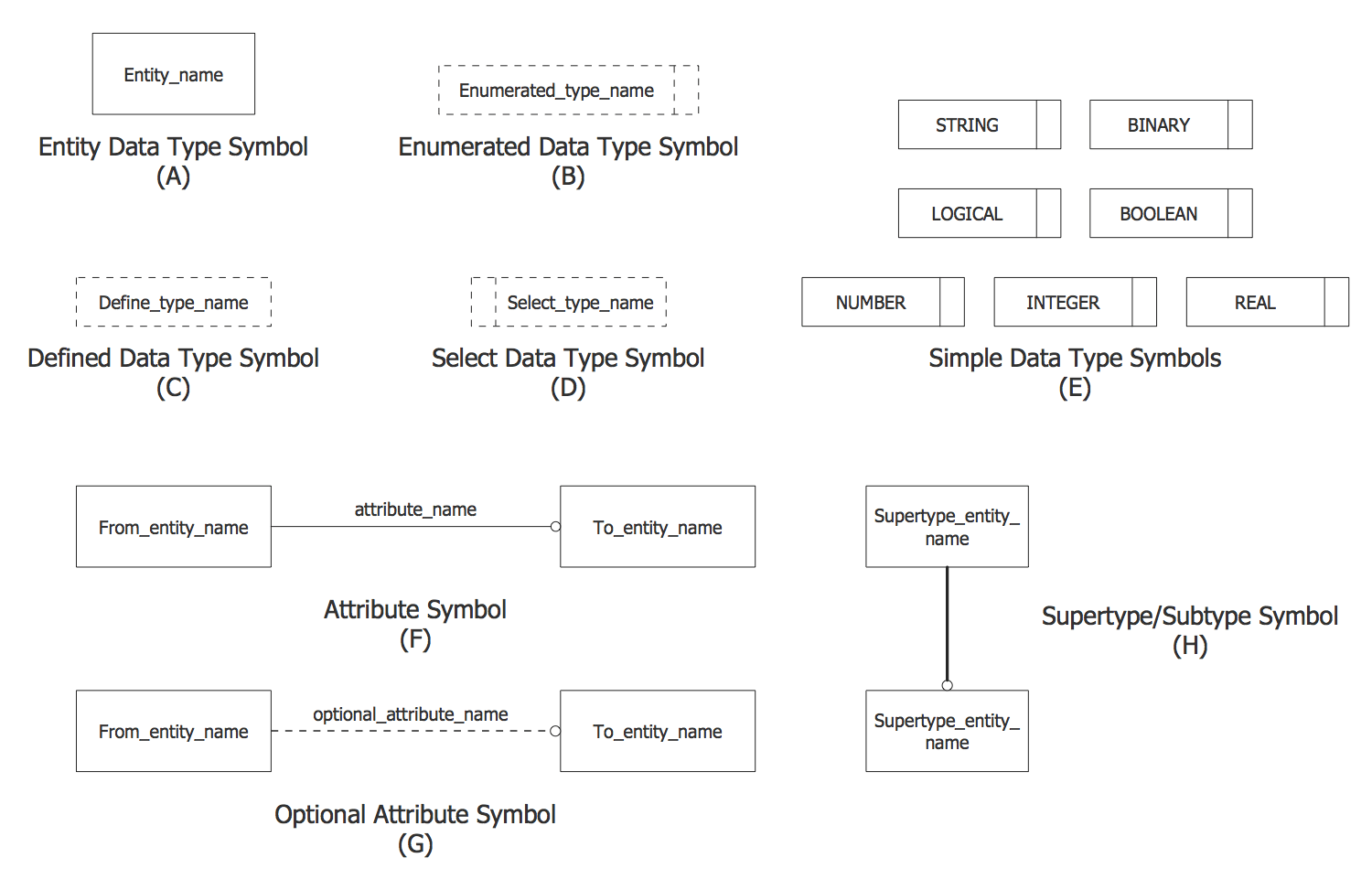All samples are copyrighted CS Odessa's.

Usage of them is covered by Creative Commons “Attribution Non-Commercial No Derivatives” License.

The text you can find at: https://creativecommons.org/licenses/by-nc-nd/3.0